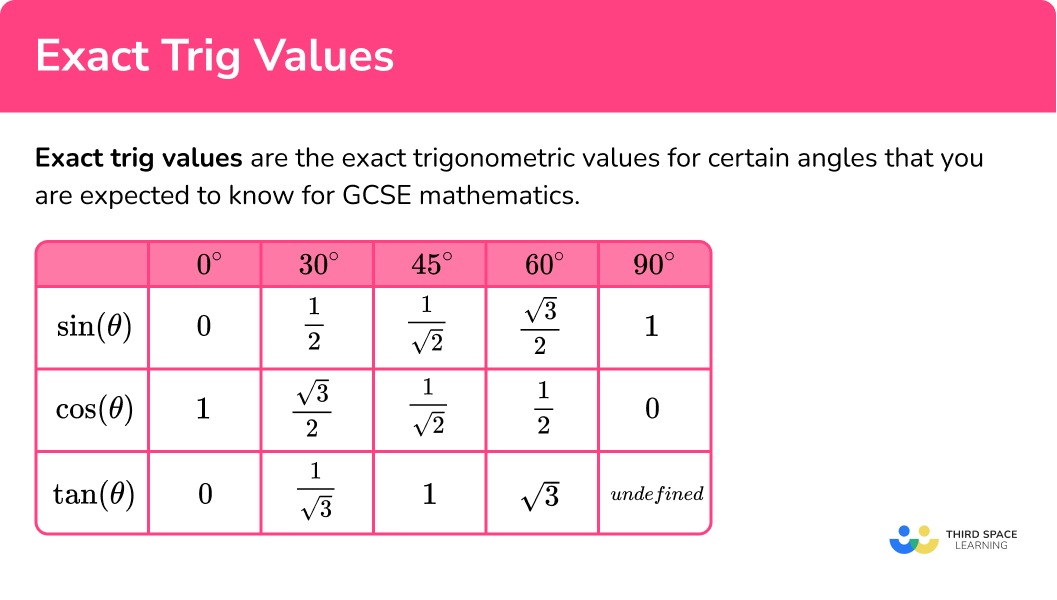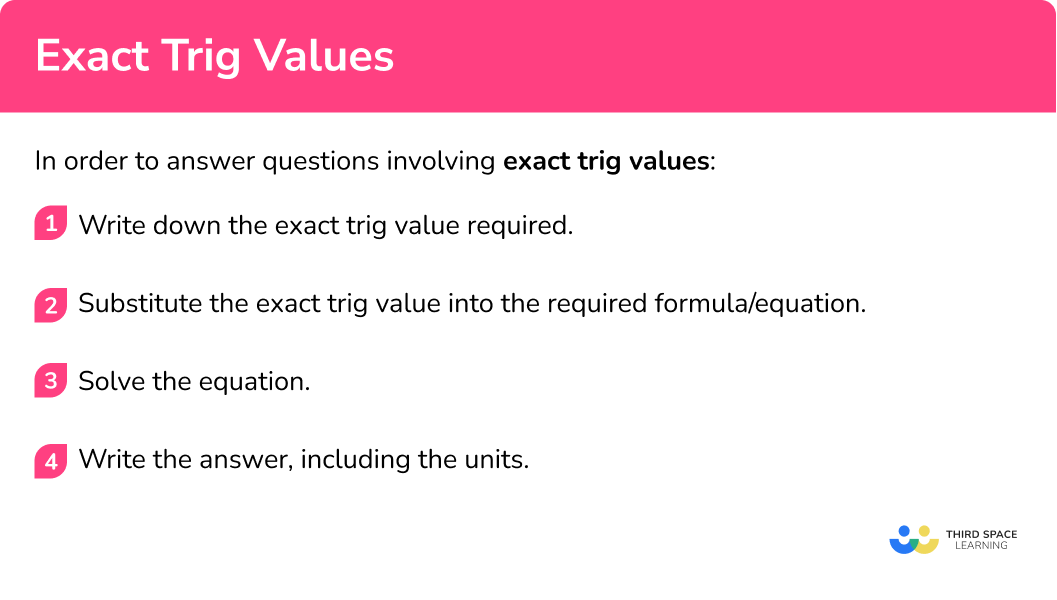# Exact Trig Values

Here we will learn about exact trig values, including what they are, how we can derive them promptly, and how we can use them to answer questions using trigonometry.

There are also exact trig values worksheets based on Edexcel, AQA and OCR exam questions, along with further guidance on where to go next if you’re still stuck.

## What are exact trig values?

Exact trig values are the exact trigonometric values for certain angles that you are expected to know for GCSE mathematics.

In trigonometry at GCSE there are three trigonometric ratios that we use, sine, cosine and tangent, though we write them as sin, cos and tan.  These trigonometric ratios show a relationship between an angle in a right-angled triangle and its side lengths.

The angle in the right-angled triangle is often labelled with a \theta (a Greek letter, ‘theta’).

\sin(\theta)=\frac{\text{Opposite}}{\text{Hypotenuse}}

Step-by-step guide: SOHCAHTOA

### What are exact trig values?## Exact trigonometric ratios

We use the three trigonometric ratios; sine, cosine, and tangent to calculate angles and lengths in right angled triangles. We can represent trigonometric ratios for the angles 30^\circ, 45^\circ, 60^\circ and 90^\circ all have exact trigonometric ratios.

We can use these exact trigonometric ratios to find lengths and angles in right angled triangles without using a calculator.

E.g.

Write down the exact value of cos 60.

\cos 60 = \frac{1}{2}

E.g.

Find the exact value of \sin 30 + \cos 60.

\sin 30 = \frac{1}{2}

\cos 60 = \frac{1}{2}

\sin 30 + \cos 60 = \frac{1}{2}+\frac{1}{2}=1

E.g.

Find the exact value of x.

Method 1

\sin 30 = \frac{5}{x}

\sin 30 =\frac{1}{2}

x = 5 ÷ \frac{1}{2} = 10cm

Method 2

\cos 60 = \frac{5}{x}

\cos 60 =\frac{1}{2}

x = 5 ÷ \frac{1}{2} = 10cm

### Deriving the exact trig values

Using the two right-angled triangles below, we can determine all of the exact trigonometric values we need.### Triangle A (sometimes referred to as the 30-60 triangle)

Triangle A is half of an equilateral triangle with a side length of 2 .

We know that SOHCAHTOA is an abbreviation for the three trigonometric ratios.

Using these three ratios in the 30-60 triangle, we can determine the exact values for sin/cos/tan of 30^{\circ} , and 60^{\circ} .\text{As }\sin(\theta)=\frac{\text{Opp}}{\text{Hyp}}, \ \sin 30=\frac{1}{2}\text{As }\sin(\theta)=\frac{\text{Opp}}{\text{Hyp}}, \ \sin 60=\frac{\sqrt{3}}{2}### Triangle B (sometimes referred to as the 45-45 triangle)

Triangle B is a right-angled isosceles triangle.

Using the three trig ratios in the triangle, we can determine the exact values for sin/cos/tan of 45^{\circ} .\text{As }\sin(\theta)=\frac{\text{Opp}}{\text{Hyp}}, \ \sin 45=\frac{1}{\sqrt{2}}=\frac{\sqrt{2}}{2}\text{As }\cos(\theta)=\frac{\text{Adj}}{\text{Hyp}}, \ \cos 45=\frac{1}{\sqrt{2}}=\frac{\sqrt{2}}{2}\text{As }\tan(\theta)=\frac{\text{Opp}}{\text{Adj}}, \ \tan 45=\frac{1}{1}=1

For sin/cos/tan of 0^{\circ} and 90^{\circ} , we can use the unit circle.

Step-by-step guide: The unit circle (coming soon)

The values for sin/cos/tan of 0^{\circ} and 90^{\circ} , are as follows:

### Summary

Here is a table of the exact trig values you are expected to learn:

Note: \tan 90 is undefined.

Some of the exact values are written with a square root symbol. These numbers are called surds.  (In mathematics surds tend to be written so there are no square roots as the denominators. This is known as rationalising surds and is covered in Higher GCSE maths.) Here, we have written the non-rationalised form and rationalised form.

## How to answer questions involving exact trig values

In order to answer questions involving exact trig values:

1. Write down the exact trig value required.
2. Substitute the exact trig value into the required formula/equation.
3. Solve the equation.
4. Write the answer, including the units.

### How to answer questions involving exact trig values## Exact trig values examples

### Example 1: stating the value

Write down the exact value of \cos 30 .

1. Write down the exact trig value required.

We need the exact value of \cos 30

2 Substitute the exact trig value into the required formula/equation.

For this question, we do not have an equation to solve so we can move on to Step 4 .

3Solve the equation.

Move on to step 4 .

4Write the answer, including the units.

There are no defined units for this question so we can simply state the exact trig value:

\cos 30=\frac{\sqrt{3}}{2}

### Example 2: stating the value

Write down the exact value of \sin 90

Write down the exact trig value required.

Substitute the exact trig value into the required formula/equation.

Solve the equation.

Write the answer, including the units.

### Example 3: using the exact values

Write down the exact value of \cos 90+\tan 45

Write down the exact trig value required.

Substitute the exact trig value into the required formula/equation.

Solve the equation.

Write the answer, including the units.

### Example 4: using the exact values

Write down the exact value of \sin 45+\tan 60 . Express your answer as a single fraction with a rationalised denominator in its simplest form.

Write down the exact trig value required.

Substitute the exact trig value into the required formula/equation.

Solve the equation.

Write the answer, including the units.

### Example 5: using an exact value to find a missing side

Calculate the length of the side x .

Write down the exact trig value required.

Substitute the exact trig value into the required formula/equation.

Solve the equation.

Write the answer, including the units.

### Example 6: using an exact value to find a missing side

Calculate the exact length of side x .

Write down the exact trig value required.

Substitute the exact trig value into the required formula/equation.

Solve the equation.

Write the answer, including the units.

### Common misconceptions

• Exact values

There is no need to write your answer as a decimal and round.  It would no longer be an exact value.

• Using exact values

If you have been given the answer, you need to make it clear that you have used the exact trig value in your workings.

• Remembering exact sin values

The exact sin values can be difficult to remember, but there is a pattern.  Here it is.

These do simplify to the familiar, exact trig values.

• Remembering exact cos values

The exact cos values can be difficult to remember, but there is a pattern.  Here it is.

These do simplify to the familiar, exact trig values.

### Practice exact trig values questions

1. What is the exact value of \cos 60 :

\frac{\sqrt{3}}{2}\frac{1}{2}1\frac{\sqrt{2}}{2}\cos 60=\frac{1}{2}2. What is the exact value of \tan 30 :

\frac{\sqrt{3}}{3}01\sqrt{3}\tan 30 =\frac{1}{\sqrt{3}}=\frac{\sqrt{3}}{3}3. What is the exact value of \cos 0+\sin 30 ?

1\frac{1+\sqrt{3}}{2}1\frac{1}{2}\frac{1}{2}\cos 0 +sin 30=1+\frac{1}{2}=1\frac{1}{2}4. What is the exact value of \cos 45+\sin 60 ?

\frac{\sqrt{3}+\sqrt{2}}{3}\frac{1+\sqrt{2}}{2}\frac{1+\sqrt{3}}{2}\frac{\sqrt{3}+\sqrt{2}}{2}\cos 45+sin 60=\frac{\sqrt{3}}{2}+\frac{1}{2}=\frac{\sqrt{3}+\sqrt{2}}{2}5. Calculate the exact value of side x .4.5 \text{ cm}3 \text{ cm}2\sqrt{3} \text{ cm}3\sqrt{2} \text{ cm}We need to label the triangle.Then we need to be using \co 60=\frac{1}{2}

\begin{aligned} \cos(\theta)&= \frac{\text{Adjacent}}{\text{Hypotenuse}} \\\\ \frac{1}{2}&= \frac{x}{6} \end{aligned}

Multiplying both sides of the equation by 6 , we have

\begin{aligned} x&=6\times \frac{1}{2} \\\\ x&=3 \text{ cm} \end{aligned}

6. Calculate the exact value of side x .4\sqrt{3} \text{ cm}\frac{\sqrt{3}}{4} \text{ cm}(4+\sqrt{3}) \text{ cm}4.3 \text{ cm}We need to label the triangle.Then we need to be using \tan 30=\frac{1}{\sqrt{3}}=\frac{\sqrt{3}}{3}

\begin{aligned} \tan(\theta) &= \frac{\text{Opposite}}{\text{Adjacent}} \\\\ \frac{\sqrt{3}}{3} &= \frac{x}{12} \\\\ x &= 12 \times \frac{\sqrt{3}}{3} \\\\ x &= 4 \sqrt{3} \text{ cm} \end{aligned}

### Exact trig values GCSE questions

1. Circle the value of \cos 90 .

(1 mark)

0

(1)

2. Work out the exact value of 8 \sin 45 .

(2 marks)

8\times \frac{\sqrt{2}}{2}

(1)

4\sqrt{2}

(1)

3. Use trigonometry to show that the length of side BC of this triangle is 10 \ cm .(3 marks)

\sin(\theta)=\frac{\text{Opposite}}{\text{Hypotenuse}}

(1)

\frac{1}{2}=\frac{5}{x}

(1)

x=5\times{2}=10

(1)

4. Here is an equilateral triangle with sides 10 \ cm .

Use trigonometry to show that the perpendicular height of this triangle is 5\sqrt{3}\text{ cm} .

(3 marks)Interior angle labelled 60^{\circ}

(1)

\sin 60=\frac{\sqrt{3}}{2}

(1)

10\times\frac{\sqrt{3}}{2}=5\sqrt{3}

(1)

## Learning checklist

You have now learned how to:

• Know the exact values of \sin \; \theta and \cos \; \theta for \theta = 0^{\circ}, 30^{\circ}, 45^{\circ}, 60^{\circ} and 90^{\circ}
• Know the exact value of \tan \; \theta for \theta = 0^{\circ}, 30^{\circ}, 45^{\circ}, 60^{\circ}
• Write down the exact trig values for certain angles
• Use the exact trig values to solve problems

## The next lessons are

### Did you know?

The exact values of tan can be found by dividing the values of sin by the values of cos.

## Still stuck?

Prepare your KS4 students for maths GCSEs success with Third Space Learning. Weekly online one to one GCSE maths revision lessons delivered by expert maths tutors.

Find out more about our GCSE maths tuition programme.